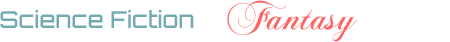## Answerer quality: Users with Top Answer Effectiveness (accepted percentage times quantity)

6

(click on this box to dismiss)

Displays the top X users according to their accepted answer percentage multiplied by their total number of answers, within the group of users with more than Y answers in total. Looks only at open, non-CW questions and answers. This is an excellent metric of *answerer quality*; users that not only produce answers in quantity, but of consistently high quality as well.Q&A for science fiction and fantasy enthusiasts

``````-- Users with Top Answer Effectiveness (accepted percentage times quantity)
-- Displays the top X users according to their accepted answer percentage
-- multiplied by their total number of answers, within the group of
-- users with more than Y answers in total.
-- Looks only at open, non-CW questions and answers.

SELECT TOP ##MaxUsers##
Sum(CASE q.AcceptedAnswerId WHEN a.Id THEN 1 ELSE 0 END) AS [Accepted Answers],
Round(Sum(CASE q.AcceptedAnswerId WHEN a.Id THEN 1 ELSE 0 END) * 100.0 / Count(a.Id), 1) AS [Percentage Accepted],
CAST(Sum(CASE q.AcceptedAnswerId WHEN a.Id THEN 1 ELSE 0 END) AS FLOAT) * (CAST(Sum(CASE q.AcceptedAnswerId WHEN a.Id THEN 1 ELSE 0 END) AS FLOAT) / Count(a.Id)) AS Weighting
FROM    Posts AS a
JOIN Posts AS q
ON a.ParentId = q.Id
WHERE
q.postTypeId = 1
AND a.postTypeId = 2
GROUP BY
a.OwnerUserId
HAVINGHold tight while we fetch your results